# Motorcyclist

From Trutnov, the motorcyclist started at an average speed of 60km/hour. At 12.30hrs the passenger car was started at a speed of 80km/hour. How many hours and at what distance from Trutnov will car catch a motorcycle?

Result

t =  17 h
s =  360 km

#### Solution:Leave us a comment of example and its solution (i.e. if it is still somewhat unclear...):

Showing 0 comments:Be the first to comment!#### To solve this example are needed these knowledge from mathematics:

Do you have a linear equation or system of equations and looking for its solution? Or do you have quadratic equation? Do you want to convert length units?

## Next similar examples:

1. Truck and car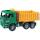At 6h45min the truck started at a speed of 36 km/h. At 7h30m behind it a passenger car. Find the average speed of passenger car if it will catch truck 72 km away from the factory.
2. Train expres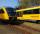At 8.00 strarted from Bratislava to Poprad express Tatry. Cities are distant 340 kilometers. At the same time started from Poprad express Danube to Bratislava. Express Tatry's average speed is 80 km/h, Danube 90 km/h. At what distance from Poprad trains w
3. Pedestrian and the cyclist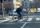Pedestrian and cyclist went against each from the two cities that are distant 40 km. Pedestrian started 30 minutes before the cyclist and walk an average speed of 5 km/h. What speed went cyclist when met after 120 minutes from the start of the pedestrian?
4. Two trains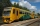From station A, the freight train traveled at a speed of 40 km/h in 9h. When he drove 15km, the fast train started from station A in the same direction at a speed of 70km/h. When will it the freight train catch up?
5. Cars 6At 9:00 am two cars started from the same town and traveled at a rate of 35 miles per hour and the other car traveled at a rate of 40 miles per hour. After how many hours will the cars be 30 miles apart?
6. Cruise liner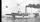Cruise liner runs along the river between points A and B. The journey downstream takes 40 minutes 1 hour upriver. The flow rate of the river is 3 km/h. What is the speed of cruise liner?
7. Cyclist vs boat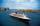The cyclist wants to ride a short distance by boat, but when he stops at the pier, the boat does not pick up passengers yet and is preparing to leave. The cyclist decides that the boat will catch up at the next stop. The stop is 12km far along the water an
8. Round-tripA woman works at a law firm in city A which is about 50 miles from city B. She must go to the law library in city B to get a document. Find how long it takes her to drive​ round-trip if she averages 40 mph.
9. Simplify 2Simplify expression: 5ab-7+3ba-9
10. Greg and BillGreg is 18 years old. He is 6 less than 4 times Bill's age. How old is Bill?
11. GivenGiven 2x =0.125 find the value of x
12. Cenda and Pepa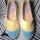Cenda and Pepa went to the event. Cenda started alone. Canda started after him for 20 minutes. How long took Cenda to catch him? Cenda traveling at 15 km/h, and Pepa traveling at 25 km/h.
13. Bob traveled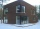Bob traveled 20 meters to Dave's house. He arrived in 3 minutes. What was his average speed for the trip.
14. Six yearsIn six years Jan will be twice as old as he was six years ago. How old is he?
15. EquationSolve the equation: 1/2-2/8 = 1/10; Write the result as a decimal number.
16. Unknown numberIdentify unknown number which 1/5 is 40 greater than one tenth of that number.
17. Simple equationSolve for x: 3(x + 2) = x - 18